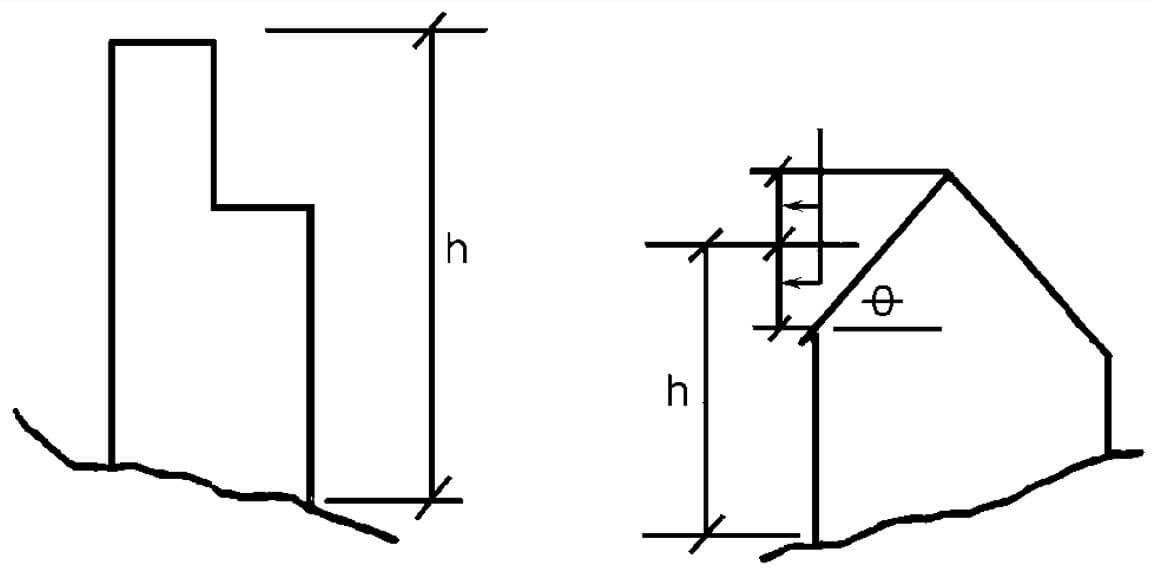# Mean Roof Height (ASCE 7)##### Per ASCE 7, the Mean Roof Height (h) is defined as the average of the roof eave height and the height to the highest point on the roof surface, except that, for roof angles of less than or equal to 10°, the mean roof height is permitted to be taken as the roof eave height.

Visually, AAMA TIR A15-14 illustrates the mean roof height as per the image below, where h on the sloped roof is = (ridge height + eave height) /2

Eave height is the distance from the ground surface adjacent to the building to the roof eave line at a particular wall. If the height of the eave varies along the wall, the average height shall be used.It is noted that the mean roof height affects only the negative components and cladding wall pressures of ASCE 7.  Positive wall pressures may use the elevation of the opening in question.

Our online components and cladding wind pressure calculator uses this mean roof height in calculating design pressures for building openings and is free to use.

In the illustration above. if the angle shown above (zero with the line through) is greater than 10 degrees.  If it’s less than 10 degrees do not use the mean, use the HIGHEST ROOF HEIGHT
(source: AAMA TIR-A15-14)

Why don’t they just call it the average roof height you may ask?  the term ‘mean’ indicates the center of 2 data points whereas average could span over many data points, fun fact!

Last Update: March 28, 2021

March 27, 2021